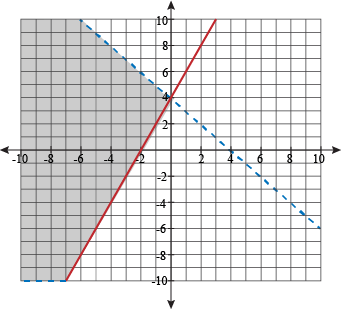# What Do You Know About Inequalities (Systems & Graphs)?

10 Questions | Total Attempts: 108SettingsIt's a mathematical topic that's pretty simple to comprehend. At least we think so! In light of that, how about some quizzes to tease your brain with?

• 1.
What do you know about the definition?
• A.

Graphs are used to show the solutions

• B.

There are three axes

• C.

All solutions must be zero

• D.

There's no need for any axis.

• 2.
How many axis/axes do you need at the basic level?
• A.

5

• B.

1

• C.

2

• D.

4

• 3.
What's the function of the boundary line?
• A.

It acts as the boundary of the Y-axis only

• B.

It divides the plane into half planes

• C.

It's the first answer after calculating the axes

• D.

There's nothing like boundary line

• 4.
Which one is feasible under this topic?
• A.

• B.

• C.

>>=

• D.

=>

• 5.
How many points (i.e 2,0,5) does two axes need in inequalities?
• A.

3.5

• B.

1

• C.

3

• D.

2

• 6.
Which one means “Greater Than” in the following?
• A.

>

• B.

• C.

=

• D.

^

• 7.
What does “Greater Than” change to when you divide through by negative (minus)?
• A.

Less than

• B.

Remains greater than

• C.

Less than or equal to

• D.

Equal to

• 8.
Do you think degrees are utilized when calculating inequalities at the lowest level?
• A.

Up to 360 degrees

• B.

Capped off at 180 degrees

• C.

None

• D.

Only when calculating the intersection box

• 9.
Which point is shaded on the graph?
• A.

Opposite of the solution area

• B.

Solution area

• C.

No area

• D.

The Y-axis

• 10.
Why is the solution area shaded?
• A.

• B.

To indicate that it's useless

• C.

To clearly identify which part is the solution

• D.

Aesthetic appeal only

Related TopicsBack to top